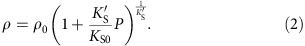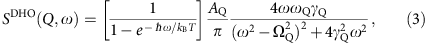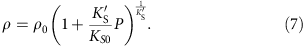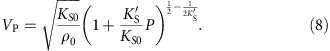## Introduction

Sound velocity and density are important observational constraints on the chemical composition of the Earth’s core. While properties of solid iron alloys have been extensively examined by laboratory studies to core pressures (>136 GPa)1,2,3, little is known for liquid alloys because of experimental difficulties. The core is predominantly molten, and the longitudinal wave (P-wave) velocity of liquid iron alloy is the key to constraining its composition. However, previous static high-pressure and -temperature (P–T) measurements of liquid iron alloys were performed only below 10 GPa using large-volume presses4,5,6. Shock wave experiments have been carried out at much higher pressures but only along a specific Hugoniot P–T path7,8.

Carbon is one of the possible light alloying components in the core because of its high cosmic abundance and strong chemical affinity with liquid iron9. Its high metal/silicate partition coefficients indicate that thousands of parts per million to several weight percent of carbon could have been incorporated into the core during its formation9,10,11. In addition, recent experimental and theoretical studies12,13 have suggested that solid Fe7C3 may explain the properties of the inner core, in particular its high Poisson's ratio14,15, supporting the presence of carbon in the core.

In this study, we determine the P-wave velocity (VP) (equivalent to bulk sound velocity, VΦ, in a liquid) of liquid Fe84C16 at high P–T based on inelastic X-ray scattering (IXS) measurements. Combined with its density data at 1 bar (ref. 16) both velocity and density (ρ) profiles of liquid Fe84C16 along adiabatic compression are obtained. They are compared with seismological observations, indicating that both VP and ρ in the Earth’s outer core are not explained simultaneously by liquid Fe–C.

## Results

### Longitudinal wave velocity measurements

We collected the high-resolution IXS spectra from liquid Fe84C16 (4.0±0.3 wt.% carbon) at static high P–T using both resistance- and laser-heated diamond-anvil cells (Methods; Fig. 1). The starting material was synthesized beforehand as a mixture of fine-grained Fe and Fe3C at 5 GPa and 1,623 K in a multi-anvil apparatus. Experimental P–T conditions were well above the eutectic temperature in the Fe–Fe3C binary system (Supplementary Fig. 1). The carbon concentration in the eutectic liquid is known to be 3.8–4.3 wt.% at 1 bar to 20 GPa (ref. 17), almost identical to the composition of our sample. Above 20 GPa, we heated the sample to temperatures comparable or higher than the melting temperature of Fe3C, a liquidus phase in the pressure range explored, assuring a fully molten sample. The molten state of the specimen was carefully confirmed, before and after the IXS measurements, by the absence of diffraction peaks from the sample (Fig. 2). We sometimes, depending on a sample volume, were also able to observe the diffuse diffraction signal typical of a liquid.

The VP of liquid Fe84C16 was determined between 7.6 and 70 GPa (Fig. 3 and Supplementary Table 1) from dispersion curves for a range of momentum transfer (Fig. 4). It was found to be 15–30% smaller than that of solid Fe (refs 3, 18, 19, 20) and Fe3C (refs 21, 22, 23; note that a starting material in the present experiments was a mixture of these solid phases) (Fig. 5), confirming that we measured a liquid sample. The velocities of a fictive solid Fe84C16 alloy are also estimated assuming a linear velocity change between Fe (ref. 24) and Fe3C (ref. 23) indicating that VP drops by 13% upon melting at 2,300 K, a eutectic temperature at 45 GPa (ref. 17). Such a velocity change is comparable to that expected for pure Fe. The difference in VΦ between solid and liquid Fe84C16 is very small (1.8%). On the other hand, the VP of our liquid Fe84C16 sample is 3–14% faster at 8–70 GPa than that of liquid Fe determined by shock-wave study8 (Fig. 3).Figure 5: Comparison of velocities between liquid and solid Fe–C alloys.

Earlier ultrasonic measurements performed below 10 GPa reported a change in VP by <2–3% per 1,000 K for liquid Fe–S alloys4,5. Theoretical calculations25,26,27 and shock compression data8 on liquid Fe and Fe–S alloy demonstrated even smaller effects above 100 GPa (<0.5% by 1,000 K). It is therefore very likely that the VP of liquid Fe84C16 is also not sensitive to temperature with the temperature effect much smaller than the uncertainty in the present velocity determinations (±3%).

### Thermodynamical equation of state

VP of a liquid can be described using the Murnaghan equation of state4 (Methods) as;where KS and K′S are adiabatic bulk modulus and its pressure derivative, respectively (zero subscripts denote values at 1 bar and T=T0). Here, consistent with the discussion above, we neglect the temperature dependence of our VP data, while ρ0 is taken to be temperature dependent16 (Methods). We fit equation (1) to our PVP data for liquid Fe84C16 and find KS0=110±9 GPa and KS=5.14±0.30 when T0=2,500 K (Supplementary Table 2 and Supplementary Fig. 2). The choice of T0 and, accordingly, the variation in ρ0 practically changed KS0 and KS0 as (∂KS0/∂T)=−9.4 × 10−3 GPa K−1 and (∂KS0/∂T) =−2.7 × 10−4 K−1. Our value for KS0 is similar to that for liquid iron8 but for KS is higher than that for pure iron, KS=4.7. This suggests that liquid Fe84C16 becomes progressively stiffer than liquid Fe with increasing pressure. We also found VP0=4,121±177 m s−1 for liquid Fe84C16 from KS0 and ρ0, in good agreement with a previous study28 of liquid Fe86C14 at 1 bar (4,050 m s−1) and faster than VP0=3,860 m s−1 for liquid Fe (ref. 8).

To compare the present results with earlier density measurements of liquid Fe–C alloys at high pressure29,30 the isothermal bulk modulus for liquid Fe84C16 is estimated to be KT0=100 (82) GPa at 1,500 K (2,500 K) from our determination of KS combined with Grüneisen parameter γ0=1.74 (ref. 8) and thermal expansion coefficient16 (Methods). When applying CP/CV=1.125 at 1,820 K for liquid Fe86C14 derived from theoretical calculations31, KT0=106–98 GPa is obtained at the same temperature range. These KT0 values for liquid Fe84C16 are similar to KT0=95–63 GPa for liquid Fe at 1,500–2,500 K (ref. 8) On the other hand, they are significantly larger than KT0=55.4 GPa for liquid Fe86C14 at 1,500 K and KT0=50 GPa for liquid Fe75C25 at 1,973 K from previous density measurements29,30. However, the calculated density for Fe84C16 using the present EoS are in reasonable agreement with the previous density measurements of Fe75C25 (ref. 29) (Fig. 6). The disagreement of elastic parameters with such earlier experiments may be attributed either to the limited pressure range of the previous density determinations, or to a different structure or magnetic (or electronic) change in the state of the liquid Fe–C at low pressure, as has been suggested from the change in compressional behaviour of liquid Fe78C22 around 5 GPa (ref. 6). Our data were collected above 7.6 GPa, so that the physical properties of liquid Fe–C obtained here should be more applicable to the Earth’s core.

ρ of liquid Fe84C16 is then given, using the elastic parameters determined above, by;Equations (1) and (2) give the VP and ρ profiles for adiabatic compression (Methods), assuming γ0=1.74, the same as that of liquid Fe (ref. 8) (Fig. 7). We find VP=9,200 m s−1 and ρ=9.82–9.61 g cm−3 at the core-mantle boundary (CMB) for TCMB=3,600–4,300 K (refs 32, 33) This indicates that VP of liquid Fe84C16 is 19.6% faster than that of liquid Fe at the CMB8, implying that the addition of 1 at.% carbon increases the VP of liquid Fe by 1.2%. The extrapolation of the present experimental data using the Murnaghan equation of state may overestimate the VP by 2−4% at the CMB (Supplementary Note 1 and Supplementary Fig. 3), but, even if this is the case, 1 at.% carbon enhances the VP of liquid Fe by as large as 0.8%. Indeed, the effect of carbon is much larger than a recent theoretical prediction of only 0.2% increase in velocity per 1 at.% carbon at 136 GPa (ref. 34). On the other hand, our data show that the incorporation of 1 at.% carbon reduces the density of liquid Fe by 0.6–0.7%, while theory suggested only 0.3% density reduction by 1 at.% carbon34.Figure 7: Velocity and density of liquid Fe84C16 extrapolated to core pressures.

## Discussion

We now compare the sound velocity and density of liquid Fe84C16 and liquid Fe with the seismologically based PREM model35 for the outer core (Fig. 8). The VP and ρ of liquid Fe are 4.6% slower and 10.1–8.6% denser, respectively, than the PREM at the CMB (3,600–4,300 K). To match the PREM values, considering the uncertainty of data extrapolation to higher pressures (Supplementary Note 1), only 5.2–4.0 at.% (1.2–0.9 wt.%) carbon is required to match the velocity, whereas 15.4–12.0 at.% (3.8–2.9 wt.%) carbon is necessary to account for the density. Therefore, carbon cannot be a predominant light element in the outer core.Figure 8: Effect of carbon on the velocity and density of liquid Fe at 136 GPa.

These results suggest there is <5.2 at.% (1.2 wt.%) carbon in the outer core, consistent with the previous cosmochemical and geochemical arguments. In particular, the silicate portion of the Earth exhibits much higher 13C/12C isotopic ratio than that of Mars, Vesta and chondrite meteorites, as may be attributed to a strong enrichment of 12C in core-forming metals9. The carbon isotopic fractionation that occurred during continuous core-formation process proposed previously36,37 will give a reasonable 13C/12C ratio in the silicate Earth, and yields 1 wt.% carbon in the core9. In addition, Wood et al.9 demonstrated that carbon strongly affects the chemical activity of Mo and W in liquid metal, so that their abundance in the mantle can be explained by partitioning between silicate melt and core-forming metal with 0.6 wt.% carbon. It has been repeatedly suggested that the inner core may be composed of Fe7C3, which accounts for high Poisson’s ratio observed14,15. The crystallization of solid Fe7C3 from a liquid outer core with <1.2 wt.% carbon may still be possible if sulfur is also included in the core38.

## Methods

### High P–T generation

Molten Fe–C alloy was obtained at high P–T in an external-resistance-heated (EH) or laser-heated (LH) diamond-anvil cell (DAC; Supplementary Table 1) using facilities installed at SPring-8. A disc of pre-synthesized Fe84C16 sample, 20–25 μm thick and 100–120 μm in diameter, was loaded into a hole of a rhenium gasket, together with two 12–17 μm thick single-crystal Al2O3 sapphire discs that served as both thermal and chemical insulators. The sample was compressed with 300 μm culet diamond anvils to a pressure of interest before heating.

In LH-DAC experiments, the sample was heated at high pressure from both sides by using two 100 W single-mode Yb fibre lasers (YLR-100-AC, IPG Photonics Corp.). The Gaussian-type energy distribution of the laser beam was converted into flat-top one with a refractive beam shaper (GBS-NIR-H3, Newport Corp.). A typical laser spot was 50–70 μm in diameter on the sample, much larger than X-ray beam size (17 μm). We determined temperature by a spetroradiometric method, and its variations within the area irradiated by X-rays and fluctuations during IXS measurements were <±10%. The pressure was obtained from the equation of state for Fe3C (ref. 39) from the lattice constant observed before melting at 1,800–2,500 K. Its error was derived from uncertainties in both temperature and the volume of Fe3C. A typical image of a sample recovered after the laser heating experiment at 70 GPa and 2,700 K is given in Supplementary Fig. 4.

Only run #FeC08 was conducted in an EH-DAC. The whole sample was homogeneously heated by a platinum-resistance heater placed around the diamonds. The temperature was obtained with a Pt-Rh (type-R) thermocouple whose junction was in contact with the diamond near a sample chamber. The temperature uncertainty was <20 K. We determined the pressure based on the Raman shift of a diamond anvil40 before heating at 300 K, whose uncertainty may be as much as ±20%.

### IXS measurements

The sound velocity of liquid Fe–C alloy was determined in the DAC by high-resolution IXS spectroscopy at the beamline BL35XU, SPring-8 (ref. 41). Both LH- and EH-DACs were placed into vacuum chambers to minimize background scattering by air. The measurements were carried out with 2.8 meV energy resolution using Si (999) backscattering geometry at 17.79 keV. The experimental energy resolutions were determined using scattering from Polymethyl–methacrylate. The incident X-ray beam was focused to about 17 μm size (full width at half maximum) in both horizontal and vertical directions by using Kirkpatrick–Baez mirrors42. The X-ray beam size was much smaller than heated area (50–70 μm for LH-DAC). Scattered photons were collected by an array of 12 spherical Si analyzers leading to 12 independent spectra at momentum transfers (Q) between 3.2 and 6.6 nm−1 with a resolution ΔQ 0.45 nm−1 (full width) that was set by slits in front of the analyzer array. The energy transfer range of ±30 (or −10 to ±30) meV was scanned for 1–3 h. Before and after IXS data collections, sample melting was confirmed by X-ray diffraction data (Fig. 2) that was collected, in situ, by switching a detector to a flat panel area detector (C9732DK, Hamamatsu Photonics K.K.)43.

The IXS spectra included three (sometimes five) peaks (Fig. 1) of Stokes and anti-Stokes components of the longitudinal acoustic (LA) phonon mode from the sample (sometimes also from a diamond), and a quasi-elastic contribution near zero energy transfer. These spectra were fitted with the damped harmonic oscillator (DHO) mode44 for acoustic phonon modes and with Lorenzian function for quasi-elastic peaks convolved by experimental resolution function. The DHO model function can be described as;where AQ, ΓQ, ΩQ, kB and ħ are the amplitude, width, and energy of inelastic modes, Boltzmann constant and Planck constant, respectively. In the fitting, temperature T was fixed at a sample temperature obtained by a spetroradiometric method or a thermocouple. The excitation energy modes appearing at both Stokes and anti-Stokes sides correspond to the phonon creation and annihilation, respectively. With increasing temperature, as given by the Bose function in equation (3), the intensities of such Stokes and anti-Stokes peaks become similar to each other. A symmetric shape of the present IXS spectra therefore assures that the IXS signals originated from a high-temperature area.

The peak at a finite energy transfer gives the frequency of each mode (Fig. 1). The excitation energies for the LA phonon mode of liquid Fe84C16 obtained in a pressure range of 7.6–70 GPa are plotted as a function of momentum transfer (Q) in Fig. 4. The compressional sound wave or P-wave velocity (VP) corresponds to the long-wavelength LA velocity at Q→0 limits;We made a linear fit to the data obtained at low Q below 3.5 nm−1 to determine the P-wave velocity (Supplementary Table 1), because positive dispersion can appear at higher Q>>3 nm−1 (ref. 45). For comparison, the results based on a sine-curve fit to all Q-range data, as is usually applied for polycrystalline samples in similar high-pressure IXS measurements46, are also given in Supplementary Table 1. In general, the error bars of the two determinations of VP overlap, though the sine fit to large Q does give slightly larger VP, as would qualitatively be expected from previous measurements on liquid iron47.

### Equation of state for liquid Fe84C16

We constructed an equation of state (EoS) for liquid Fe84C16 to extrapolate the present VP data and to estimate its density at the core pressure range. VP of liquid can be written as;The pressure dependence of KS is assumed to bewhere K′S is the pressure derivative of KS and pressure and subscript zero indicates a value at 1 bar. The adiabatic Murnaghan EoS can be described as (for example, ref. 4);Equation (5) is thus rewritten as;The temperature effect on ρ0 can be expressed by;The thermal expansion coefficient α is also dependent on temperature as;where a and b are constants. Previous density measurements16 of liquid Fe–C alloys at 1 bar give a=6.424 × 10−5 K−1 and b=0.606 × 10−8 K−2 for liquid Fe84C16 using ρ0=6.505 g cm−3 at T0=2,500 K as a reference. The result of fitting equation (8) to the present PVP data is given in Fig. 3.

### Isothermal bulk modulus

We estimate isothermal bulk modulus KT from isentropic bulk modulus KS in two ways. The relationship between these two is described as follows;where CP and CV are heat capacities at constant pressure and volume, respectively. Although γ for liquid Fe–C alloys is not known, γ0=1.74 has been reported for liquid Fe at 1 bar and 1,811 K (ref. 8) It is close to 1.58 for liquid Fe90O8S2 estimated from the shock compression data set48.

### Extrapolation of present data to core pressures

With the EoSs determined above (equations (7) and (8)), we extrapolate the P-wave velocity and density of liquid Fe84C16 to the core pressure range along adiabatic compression, in which temperature is given by;Assuming γ=γ0 × (ρ0/ρ), temperature is simply represented as;γ0 is fixed at 1.74 previously obtained for liquid Fe (ref. 8). Using the temperature dependence of KS0 and ρ0 shown above, we calculate density, velocity and temperature profiles along adiabatic compression with various reference temperatures at the CMB. The adiabatic compression profiles of liquid Fe84C16 for the low (T0=2,045 K and TCMB=3,600 K)32 and high (T0=2,457 K and TCMB=4,300 K)33 temperature cases are calculated in Fig. 7.